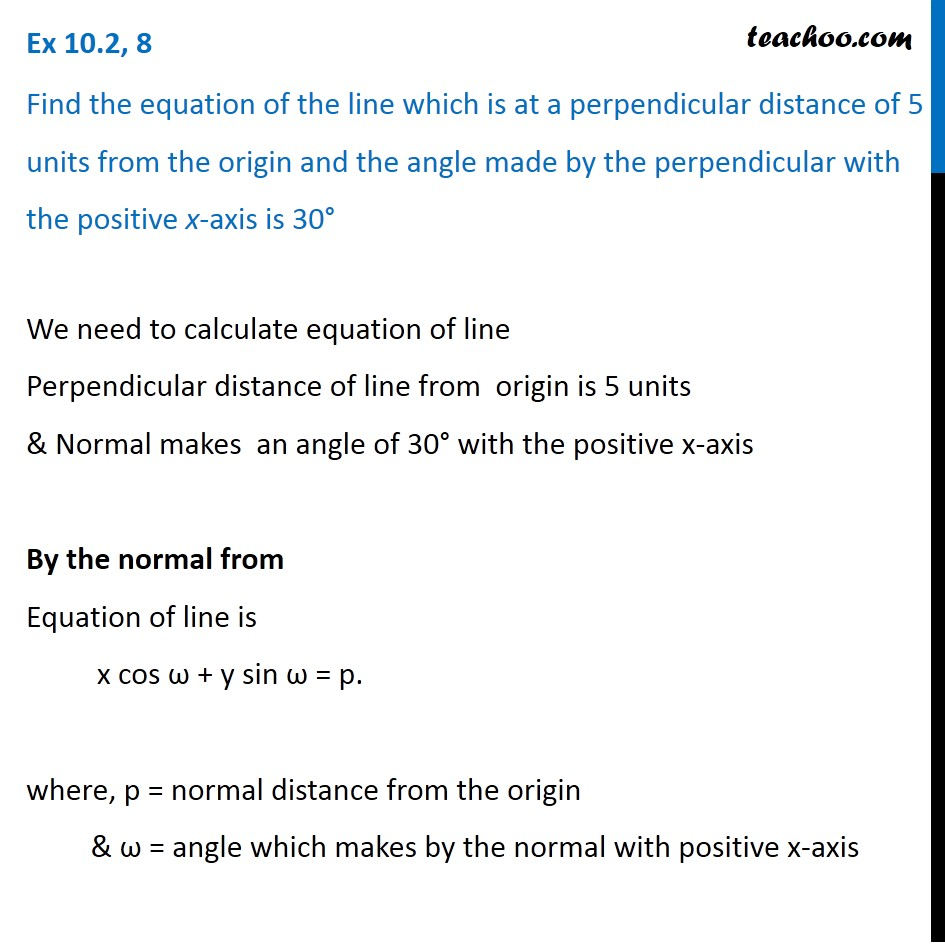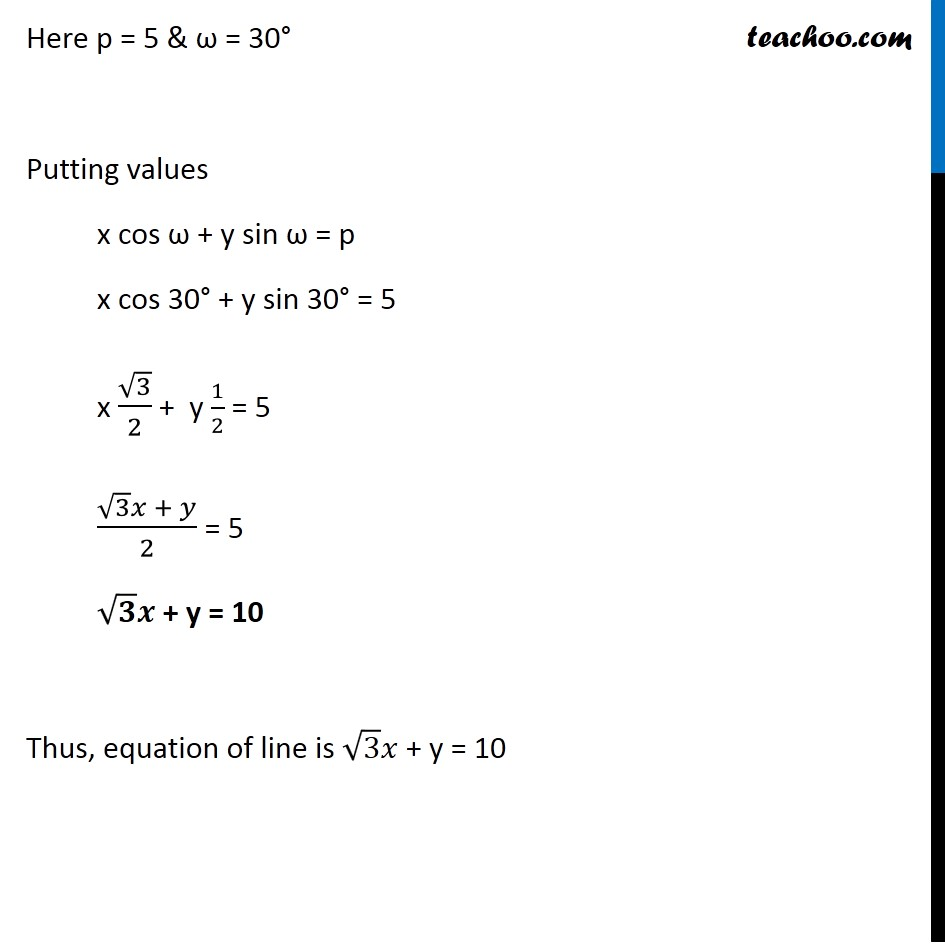Subscribe to our Youtube Channel - https://you.tube/teachoo

1. Chapter 10 Class 11 Straight Lines
2. Concept wise
3. Normal form

Transcript

Ex 10.2, 8 Find the equation of the line which is at a perpendicular distance of 5 units from the origin and the angle made by the perpendicular with the positive x-axis is 30° We need to calculate equation of line Perpendicular distance of line from origin is 5 units & Normal makes an angle of 30° with the positive x-axis By the normal from Equation of line is x cos ω + y sin ω = p. where, p = normal distance from the origin & ω = angle which makes by the normal with positive x-axis Here p = 5 & ω = 30° Putting values x cos ω + y sin ω = p x cos 30° + y sin 30° = 5 x √3/2 + y 1/2 = 5 (√3 𝑥 + 𝑦)/2 = 5 √𝟑 𝒙 + y = 10 Thus, equation of line is √3 𝑥 + y = 10

Normal form